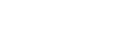# Water Velocity and limits for different design authorities

Some fire sprinkler design authorities and design standards, such as EN 12845, limit the velocity through pipes and valves in fire sprinkler systems.

This is the case with EN 12845. However, NFPA and FM do not have any restrictions on velocity. FM Global Property Loss Prevention datasheet 2-0 restricts the Hazen-Williams pressure loss equation to a velocity below 9.0 m/s (30 ft). If you exceed this velocity, use the Darcy-Weisbach pressure loss equation.

The case for limiting velocity is that the Hazen-Williams formula is less accurate outside its normal range, and the calculation loses its validity. Some authorities believe that velocity is self-limiting as pressure losses increase exponentially as velocity increases, so pipe sizes must be increased to make use of available water supply pressure. That said, we must not use the Hazen-Williams equation outside its parameters, as it is empirical (observation or experimentation). If the velocities exceed those used to determine the equation, then the calculation may not be valid.

EN 12845 limits velocity to 6 m/s through valves and flow switches and 10 m/s at any other point in the system.

Velocity in a pipe can be calculated using the following formula:-when:

v = velocity in the pipe in m/s
Q = flow of water in the pipe in L/min
d = internal diameter of the pipe in mm

The following table below lists the maximum flows in litres per minute, which can be obtained through steel pipework to EN 10255 specifications for 6 m/s and 10 m/s.### Use our online velocity calculator.

Now you have read this article and understand the importance of velocity, why not try our Pipe Velocity Calculator - Online Calculators & Tools.